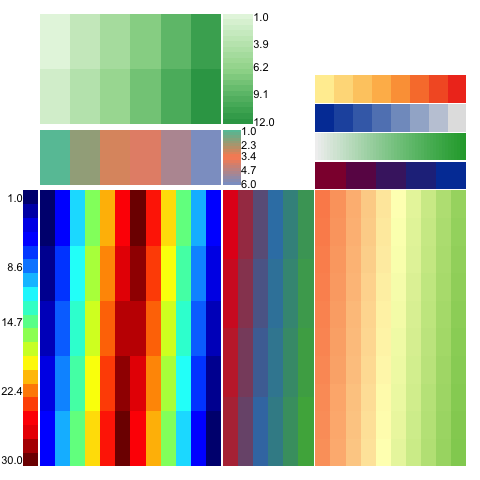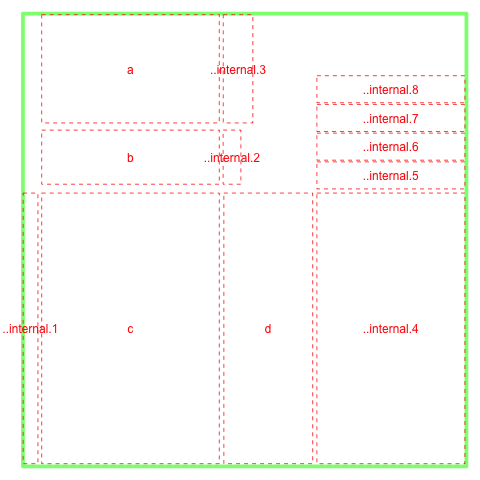# WHeatmapWHeatmap designs a set of languages and a layer system that allows arbitrary positioning of heatmaps programmatically.

It makes plotting complex heatmaps using plain English such as TopOf, RightOf and BottomLeftOf etc.

To install from github using devtools,

``````library(devtools)
install_github('zwdzwd/wheatmap')

library(wheatmap)``````

For example, the following complex layoutThis complex layout

can be generated simply by

``````WHeatmap(matrix(1:12,nrow=2), cmp=CMPar(brewer.name='Greens'), name='a') +
WHeatmap(matrix(1:6,nrow=1), Beneath(pad=0.05), cmp=CMPar(brewer.name='Set2'), name='b') +
WHeatmap(matrix(c(1:30,30:1),nrow=5), Beneath(pad=0.05), 'c', cmp=CMPar(cmap='jet')) +
WHeatmap(matrix(1:24,nrow=4), RightOf('c'), 'd', cmp=CMPar(brewer.name='Set1')) +
WLegendV('c', LeftOf('c', pad=0.01), yticklabel.side='l') +
WLegendV('b', RightOf('b', width=0.1)) +
WLegendV('a', RightOf('a')) +
WHeatmap(matrix(1:100, nrow=10), RightOf('d'), cmp=CMPar(brewer.name='RdYlGn')) +
WColorBarH(matrix(5:1), TopOf(), cmp=CMPar(colorspace.name = 'diverge_hcl')) +
WColorBarH(matrix(50:1), TopOf(), cmp=CMPar(colorspace.name = 'terrain_hcl')) +
WColorBarH(matrix(1:8), TopOf(), cmp=CMPar(colorspace.name = 'sequential_hcl')) +
WColorBarH(matrix(1:8), TopOf(), cmp=CMPar(brewer.name = 'YlOrRd'))``````

One who is really obssessed with `%>%` can do something like

``````library(magrittr)
WHeatmap(cc\$mat, name='h1') %>% add(WColorBarV(row.data, LeftOf('h1'), 'c1'))``````

The layout looks likethis

In order to cross-refer panels in the plot, one has to use a unique name. This name can be given through the `name=` option and user has to make sure the uniqueness of the names from existing objects in the plot. If not specified, wheatmap can generate a unique name and that name is visible from `ly()` function. A special `NULL` tag can be used to refer to the last plotted object.

More User guides:

## Acknowledgement

• The internal `grid.dendrogram()` function is adapted from `ComplexHeatmap` package.